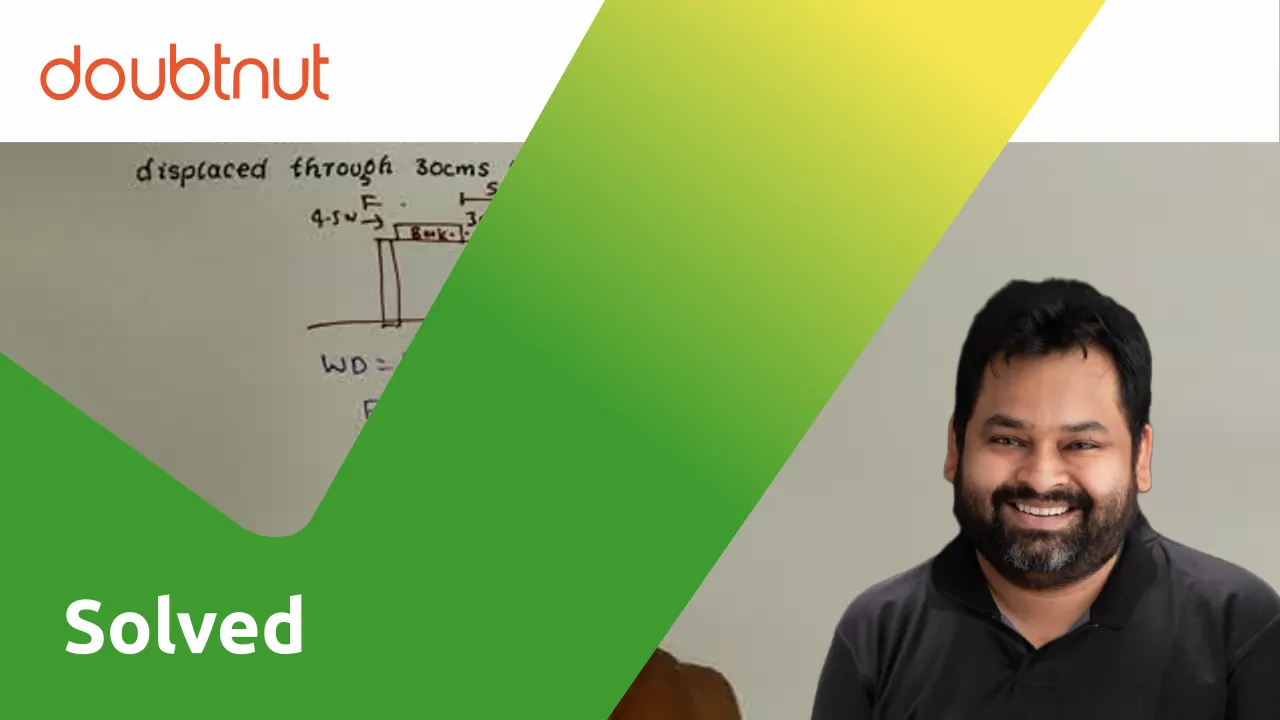Doubtnut App - Get 50 Lakh+ video solutions better than GoogleInstall Now

# A boy pushes a book kept on a table by applying a force of 4.5 N. Find the work done by the force if the book is displaced through 30 cm along the direction of push.Solution in Telugu

Text Solution
Verified by Experts

## Force applied on the book (F) = 4.5 N Displacement (s) = 30 cm = (30/100) m = 0.3 m Work done, W = Fs = 4.5 × 0.3 = 1.35 J